﻿ 基于游记文本内容的旅游场景知识图谱的构建 Construction of Tourism Scene Knowledge Graph Based on the Content of Travelogs

Hans Journal of Data Mining
Vol. 10  No. 01 ( 2020 ), Article ID: 33882 , 12 pages
10.12677/HJDM.2020.101006

Construction of Tourism Scene Knowledge Graph Based on the Content of Travelogs

Xiaohong Zhang*, Biao Ma

Department of Management, Donghua University, Shanghai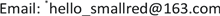Received: Dec. 18th, 2019; accepted: Jan. 2nd, 2020; published: Jan. 9th, 2020ABSTRACT

The traditional knowledge representation based on the concept of tourism ontology, which is focusing on the description of static features and lacks dynamic features. If some dynamic information hidden in the online UGC is fully utilized, such as the inheritance relationship of tourism events, group tourism Features such as emotions, can further enhance the interpretability of the tourism knowledge graph, thereby providing a more inferred knowledge graph for intelligent applications of experiential tourism. Therefore, this paper proposes a tourism scene knowledge graph (TSKG) representation model based on Bayesian network. The model uses tourism events as unit entity to obtain people’s travel emotional information from the travelogs. The sentiment difference and transition probability between destinations is used as the dynamic attribute of edges to enrich the information of edges in the graph. The experimental result shows that the LTTE recommendation model based on the TSKG performs significantly better than the benchmark algorithm TF-IDF in the tourism destination recommendation experiment.

Keywords:Knowledge Graph of Travel Scene, Bayesian Network, Tourism Emotion, Travelog Mining[目的/意义]：传统以旅游本体概念为基础的知识表示形式，侧重对静态特征的描述，缺乏对动态特征的捕捉，如若考虑融入在线UGC中的一些动态信息，如：旅游活动事件发生的顺承关系，群体旅游情绪等特征，可进一步增强旅游知识图谱的可解释性，从而为当今体验式旅游的智能应用提供更加具有可推理性的知识库。[方法/过程]：因此，本文提出一种基于贝叶斯网络的旅游场景知识图谱表示模型，该模型以旅游活动事件作为单位实体节点，从游记文本中获取人们的旅游情感信息扩充实体的动态属性，并以人们在目的地之间的转移概率和情感差值作为边的动态属性，丰富图谱中边的信息，从而提升知识图谱的可解释性。[结论]：最终实验表明，基于本文所构建的旅游场景知识图谱设计的LTTE推荐模型，在旅游目的地推荐实验中表现的效果明显优于基准算法TF-IDF。1. 引言

2. 相关工作

2.1. 旅游领域知识图谱研究现状

2.2. 游记文本挖掘研究现状

3. 旅游场景知识图谱的构建

3.1. 旅游场景知识图谱的概念模型

1) 旅游场景图谱中的实体节点N定义见如下公式：

$\text{N}=〈\text{Nid},\text{Where},\text{What},\text{How},\text{Score},\text{Text},\text{Rid}〉$ (3.2)

2) 旅游场景图谱中的旅游活动事件节点关系R定义见如下公式：

$\text{R}=〈\text{Rid},\text{RSematic},\text{RProbility},\text{RScore}〉$ (3.3)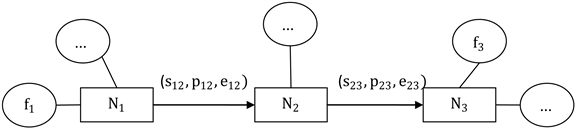Figure 1. Knowledge unit in single travel scene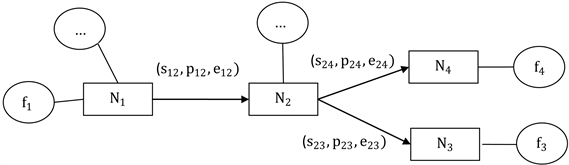Figure 2. Knowledge unit in many travel scenes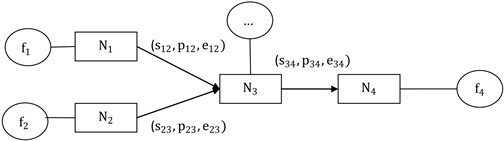Figure 3. Knowledge unit in many travel scenes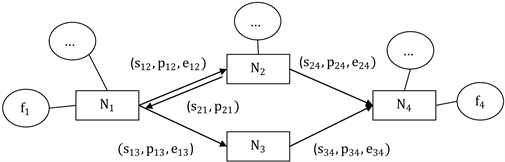Figure 4. Knowledge unit in combined travel scene

3.2. 旅游场景知识图谱的构建Figure 5. Construction logic of travel scene knowledge graph

1) 旅游目的地实体的抽取及判别

a) 基于触发词判别段落的实际描述对象

b) 结合上下文过滤干扰实体

2) 旅游目的地实体属性值的抽取

a) 旅游主题活动的抽取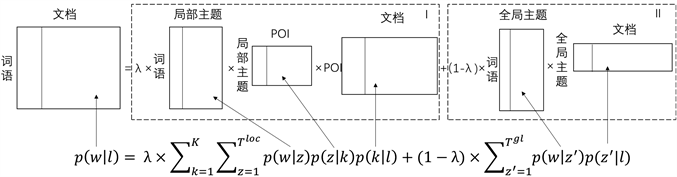Figure 6. Graph of Location-topic model

b) 旅游对象–情感词对的抽取Table 1. Extract part-of-speech patterns of topic-emotional phrases

3) 旅游目的地之间转移概率和情感差值的计算

a) 基于贝叶斯网络的转移概率的计算

$P\left({X}_{1},{X}_{2},\cdots ,{X}_{n}\right)={\prod }_{i=1}^{n}p\left({X}_{1}|{X}_{i},{X}_{2},\cdots ,{X}_{i-1}\right)$ (3.4)

b) 基于情感分析模型的情感差值计算

$Scor{e}_{k}=\lambda {\sum }_{n}E〈\text{tword},\text{sword}〉×\alpha \left(\text{nword}\right)×\beta \left(\text{dword}\right)×\gamma \left(\text{position}\right)×\delta \left(\text{rword}\right)/m$ (3.5)

$\Delta E=Scor{e}_{k}-Scor{e}_{k-1}$ (3.6)

4. 基于旅游场景知识图谱的应用

4.1. 旅游目的地实体相似度度量

$p\left(w|k\right)=\frac{{n}_{k}^{w}}{{\sum }_{{w}^{\prime }=1}^{W}{n}_{k}^{{w}^{\prime }}}$ (4.1)

$LocSim\left(PO{I}_{k},PO{I}_{k+1}\right)\stackrel{\text{def}}{=}\mathrm{exp}\left[-\tau \cdot {D}_{JS}\left({\phi }_{{k}_{1}},{\phi }_{{k}_{2}}\right)\right]\in \left(0,1\right]$ (4.2)

${D}_{JS}\left({\phi }_{{k}_{1}},{\phi }_{{k}_{2}}\right)=\frac{1}{2}{D}_{KL}\left({\phi }_{{k}_{1}}||\frac{{\phi }_{{k}_{1}}+{\phi }_{{k}_{2}}}{2}\right)+\frac{1}{2}{D}_{KL}\left({\phi }_{{k}_{2}}||\frac{{\phi }_{{k}_{1}}+{\phi }_{{k}_{2}}}{2}\right)\ge 0$ (4.3)

${D}_{KL}\left({\phi }_{{k}_{1}}||{\phi }_{{k}_{2}}\right)=\underset{i}{\sum }{\phi }_{{k}_{1}}{}_{i}\mathrm{log}\frac{{\phi }_{{k}_{1}}}{{\phi }_{{k}_{2}}}\ge 0$ (4.4)

$Score\left(k\right)=\mathrm{log}LocSim\left(k,{k}_{{\phi }_{k}}\right)+\alpha \cdot \mathrm{log}\Delta E\left(k,{k}_{{\phi }_{k}}\right)+\beta \cdot \mathrm{log}P\left(k,{k}_{{\phi }_{k}}\right)$ (4.5)

4.2. 基于旅游场景知识图谱的推荐

$Score\left(k\right)=\mathrm{log}LocSim\left(k,{k}_{{\phi }_{k}}\right)+\alpha \cdot \mathrm{log}\Delta E\left(k,{k}_{{\phi }_{k}}\right)+\beta \cdot P\left(k,{k}_{{\phi }_{k}}\right)$ (4.6)

5. 实验设计与结果分析

5.1. 实验语料预处理

5.2. 基于旅游场景知识图谱的推荐

1) 实验设计及评估指标

TCS方法：对于每个地点，将所有提及它的游记连接成一偏“大”文档，然后利用TF-IDF表示这些对应于地点的文档，最后将两个地点的相似度定义为二者对应文档的TF-IDF向量之间的余弦相似度。

a) 召回率(Recall)

$R\left({A}_{u}\right)=\frac{{A}_{u}\cap {B}_{u}}{{B}_{u}}$ (5.1)

$R\left(A\right)=\frac{1}{n}{\sum }_{u\in U}R\left({A}_{u}\right)$ (5.2)

b) Mean Reciprocal Rank (MRR)

MRR指标是在国际上对推荐算法进行评判的通用机制，用于评判推荐算法质量的高低。其评估思想是，若第一个推荐结果即与实际旅游目的地相符，则计分为1，若第二个推荐结果是正确的则计分为1/2，由此推导则第N个推荐结果与实际相符，则计分为1/n，若推荐列表中不存在，则计分为0，将所有分数进行加和即得到最终分数，计算公式如下：

$\text{MRR}=\frac{{\sum }_{n=1}^{N}\frac{1}{ran{k}_{n}}}{N}$ (5.3)

2) 实验结果分析

a) 推荐数目对推荐效果的影响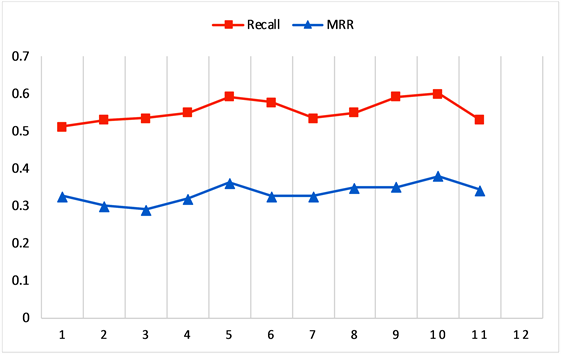Figure 7. Recall and MRR value of different recommended list numbers

b)不同推荐算法的对比实验Table 2. Evaluation index results of recommended algorithm

6. 结论

Construction of Tourism Scene Knowledge Graph Based on the Content of Travelogs[J]. 数据挖掘, 2020, 10(01): 56-67. https://doi.org/10.12677/HJDM.2020.101006

1. 1. https://www.world-tourism.org

2. 2. DERI, on Tour Ontology.

3. 3. Mili, H., Valtchev, P., Charif, Y., et al. (2011) E-Tourism Portal: A Case Study in Ontology-Driven Development. In: E-Technologies: Transformation in a Connected World, Springer, Berlin Heidelberg, 76-99.
https://doi.org/10.1007/978-3-642-20862-1_6

4. 4. 冯欣, 王成良. 本体在旅游信息系统中的应用研究[J]. 计算机与现代化, 2010(3): 128-132.

5. 5. 李艳, 王重英, 屈正庚. 基于主题词表的旅游政务系统本体构建研究[J]. 信息技术, 2015(3): 53-56.

6. 6. 奚凡. 基于情景感知的自适应旅游活动与推荐系统研究[D]: [硕士学位论文]. 上海: 东华大学, 2013.

7. 7. 杨青云, 尹鹏飞. 基于语义网的旅游信息服务平台研究[J]. 邵阳学院学报(自然科学版), 2014, 11(2): 19-24.

8. 8. Yuan, H., Qian, Y., Yang, R. and Ren, M. (2014) Human Mobility Discovering and Movement Intention Detection with GPS Trajectories. Decision Support Systems, 63, 39-51.
https://doi.org/10.1016/j.dss.2013.09.010

9. 9. Lu, X., Wang, C., Yang, J.M., et al. (2010) Photo2Trip: Generating Travel Routes from Geo-Tagged Photos for Trip Planning. In: ACM International Conference on Multimedia, ACM, New York, 143-152.
https://doi.org/10.1145/1873951.1873972

10. 10. 赵振斌, 党娇. 基于网络文本内容分析的太白山背包旅游行为研究[J]. 人文地理, 2011, 26(1): 134-139.

11. 11. 吴恒, 陈燕翎. 基于UGC文本挖掘的游客目的地选择信息研究——以携程蜜月游记为例[J]. 情报科学, 2017, 35(1): 101-105.

12. 12. Banyai, M.T.D. (2012) Evaluating Research Methods on Travel Blogs. Journal of Travel Research, 51, 267-277.
https://doi.org/10.1177/0047287511410323

13. 13. 胡乔楠. 基于旅游文记的旅游景点推荐及行程路线规划系统[D]: [硕士学位论文]. 杭州: 浙江大学, 2015.

14. 14. Hao, Q., Cai, R., Wang, X.J., et al. (2009) Generating Loca-tion Overviews with Images and Tags by Mining User-Generated Travelogues. Proceedings of the 17th In-ternational Conference on Multimedia 2009, Vancouver, British Columbia, Canada, October 19-24 2009, 801-804.

15. 15. 梁柱, 曾绍玮. 知识表示技术研究[J]. 科学咨询(决策管理), 2010(1): 52.

16. 16. 廖开际, 叶东海, 闫健峻, 等. 基于加权语义网的专家知识发现及表示方法[J]. 情报学报, 2012, 31(1): 60-64.

17. 17. 陈祖国, 李勇刚, 卢明, 陈超洋, 刘端. 基于贝叶斯概率语义网的铝电解槽况知识表示模型与约简方法[J/OL]. 控制与决策, 1-16.

18. NOTES

*通讯作者。